# Egorov generalized function algebra

(diff) ← Older revision | Latest revision (diff) | Newer revision → (diff)

Given an open subsetof, Yu.V. Egorov [a1] defined the generalized function algebra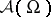as the factor algebra of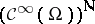modulo the ideal of sequenceswhich vanish eventually on every compact subset of. The family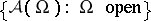provides a sheaf of differential algebras on. Convolution with a sequence of mollifiers, whereconverges to the Dirac measure, gives an imbedding of the space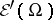of compactly supported distributions intowhich respects derivatives as well as supports. It can be extended as a sheaf morphism to an imbedding of the space of distributions.

As a generalized function algebra,can be employed to study non-linear partial differential equations. In particular, Egorov has used the algebra to construct generalized solutions to boundary value problems as well as evolution equations. In the latter case the spatial derivativemay be replaced by the difference operatorwhere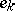denotes theth unit vector in. This way partial differential equations are approximated by ordinary difference-differential equations in the algebra.